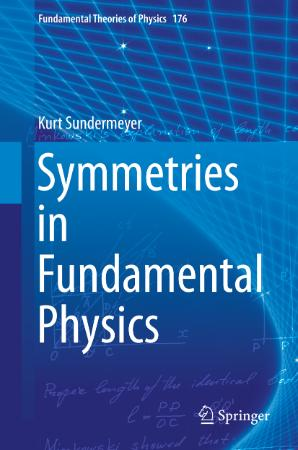## Symmetries in Fundamental Physics (Fundamental Theories of Physics)Symmetries in Fundamental Physics (Fundamental Theories of Physics)
epub | 10.54 MB | Author :Kurt Sundermeyer | Springer Netherlands, Dordrecht

Book Description :

This is a textbook that derives the fundamental theories of physics from symmetry.
It starts by introducing, in a completely self-contained way, all mathematical tools needed to use symmetry ideas in physics. Thereafter, these tools are put into action and by using symmetry constraints, the fundamental equations of Quantum Mechanics, Quantum Field Theory, Electromagnetism, and Classical Mechanics are derived.

As a result, the reader is able to understand the basic assumptions behind, and the connections between the modern theories of physics. The book concludes with first applications of the previously derived equations.

Thanks to the input of readers from around the world, this second edition has been purged of typographical errors and also contains several revised sections with improved explanations.*

Category :